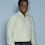# HackerRank Validating phone numbers solution in python

In this Validating phone numbers problem, You are given some input, and you are required to check whether they are valid mobile numbers.

## Problem solution in Python 2 programming.

```import re
n = int(raw_input())
for x in range(0, n):
if(re.match(r'^[7-9]{1}[0-9]{9}\n?\r?\$', raw_input())):
print "YES"
else:
print "NO"```

## Problem solution in Python 3 programming.

```# Enter your code here. Read input from STDIN. Print output to STDOUT
import re
for _ in range(int(input())):
if re.match(r'\d{9}\$',input()):
print('YES')
else:
print('NO')```

### Problem solution in pypy programming.

```import re
n = int(raw_input())
for i in range(n):
s = raw_input()
print 'YES' if re.match(r'^[7-9]\d{9}\$', s) else 'NO'```

### Problem solution in pypy3 programming.

```# Enter your code here. Read input from STDIN. Print output to STDOUT
import re
N = int(input())
for _ in range(N):
pattern = r'^\d{9}\$'
print ( 'YES' if re.match(pattern,input()) else 'NO')```

1.